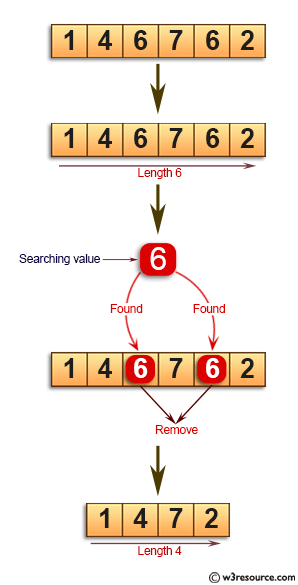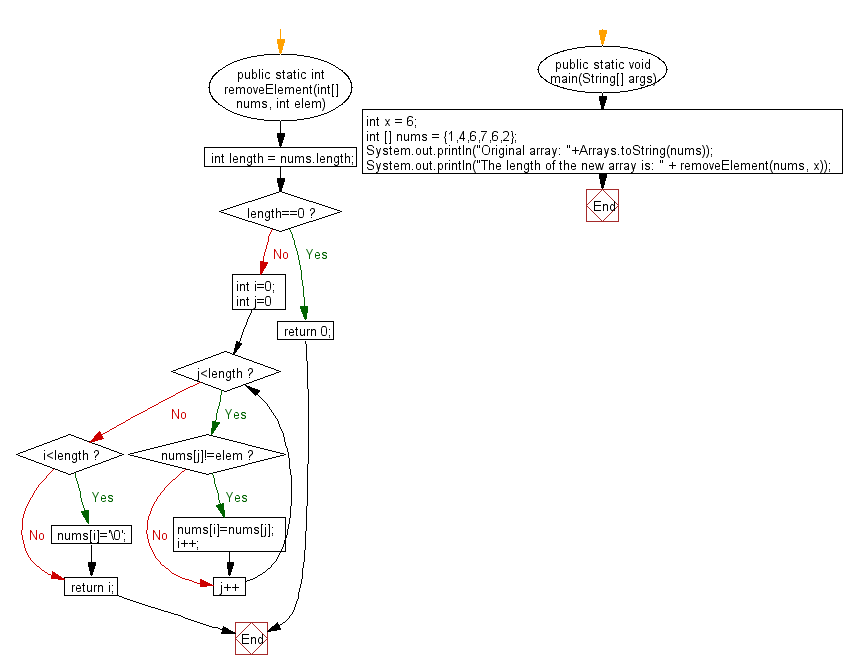﻿ Java: Remove all occurrences of a value in an array# Java Exercises: Remove all occurrences of a specified value in a given array, return the new length

## Java Basic: Exercise-144 with Solution

Write a Java program to remove all occurrences of a specified value in a given array of integers and return the new length of the array.

Pictorial Presentation:Sample Solution:

Java Code:

``````import java.util.*;
public  class Solution {
/**
*@param nums: A list of integers
*@param element: An integer
*@return: The new length after remove
*/
public static int removeElement(int[] nums, int elem) {
int length = nums.length;
if(length==0) return 0;
int i=0;
for(int j=0; j<length; j++)
{
if(nums[j]!=elem)
{
nums[i]=nums[j];
i++;
}
}
if(i<length) nums[i]='\0';
return i;
}
public static void main(String[] args) {
int x = 6;
int [] nums = {1,4,6,7,6,2};
System.out.println("Original array: "+Arrays.toString(nums));
System.out.println("The length of the new array is: " + removeElement(nums, x));
}
}
```
```

Sample Output:

```Original array: [1, 4, 6, 7, 6, 2]
The length of the new array is: 4
```

Flowchart:Java Code Editor:

What is the difficulty level of this exercise?

Test your Programming skills with w3resource's quiz.

﻿

## Java: Tips of the Day

countOccurrences

Counts the occurrences of a value in an array.

Use Arrays.stream().filter().count() to count total number of values that equals the specified value.

```public static long countOccurrences(int[] numbers, int value) {
return Arrays.stream(numbers)
.filter(number -> number == value)
.count();
}
```

Ref: https://bit.ly/3kCAgLb